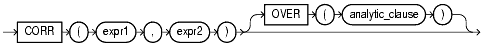## CORR

#### Syntax

corr::=Text description of corr

#### Purpose

`CORR` returns the coefficient of correlation of a set of number pairs. You can use it as an aggregate or analytic function.

Both `expr1` and `expr2` are number expressions. Oracle applies the function to the set of (`expr1`, `expr2`) after eliminating the pairs for which either `expr1` or `expr2` is null. Then Oracle makes the following computation:

```COVAR_POP(expr1, expr2) / (STDDEV_POP(expr1) * STDDEV_POP(expr2))

```

The function returns a value of type `NUMBER`. If the function is applied to an empty set, then it returns null.

 See Also: "Aggregate Functions" "About SQL Expressions" for information on valid forms of `expr`

#### Aggregate Example

The following example calculates the coefficient of correlation between the list prices and minimum prices of products by weight class in the sample view `oe.products`:

```SELECT weight_class, CORR(list_price, min_price)
FROM product_information
GROUP BY weight_class;

WEIGHT_CLASS CORR(LIST_PRICE,MIN_PRICE)
------------ --------------------------
1                  .99914795
2                 .999022941
3                 .998484472
4                 .999359909
5                 .999536087
```

#### Analytic Example

The following example returns the cumulative coefficient of correlation of monthly sales revenues and monthly units sold from the sample tables `sh.sales` and `sh.times` for year 1998:

```SELECT t.calendar_month_number,
CORR (SUM(s.amount_sold), SUM(s.quantity_sold))
OVER (ORDER BY t.calendar_month_number) as CUM_CORR
FROM sales s, times t
WHERE s.time_id = t.time_id AND calendar_year = 1998
GROUP BY t.calendar_month_number
ORDER BY t.calendar_month_number;

CALENDAR_MONTH_NUMBER   CUM_CORR
--------------------- ----------
1
2          1
3 .994309382
4 .852040875
5 .846652204
6 .871250628
7 .910029803
8 .917556399
9 .920154356
10  .86720251
11 .844864765
12 .903542662

```

Correlation functions require more than one row on which to operate, so the first row in the preceding example has no value calculated for it.